# 推荐系统遇上深度学习(六)--PNN模型理论和实践

www.jianshu.com/p/6f1c2643d…

www.jianshu.com/p/77719fc25…

# 1、原理

PNN，全称为Product-based Neural Network，认为在embedding输入到MLP之后学习的交叉特征表达并不充分，提出了一种product layer的思想，既基于乘法的运算来体现体征交叉的DNN网络结构，如下图：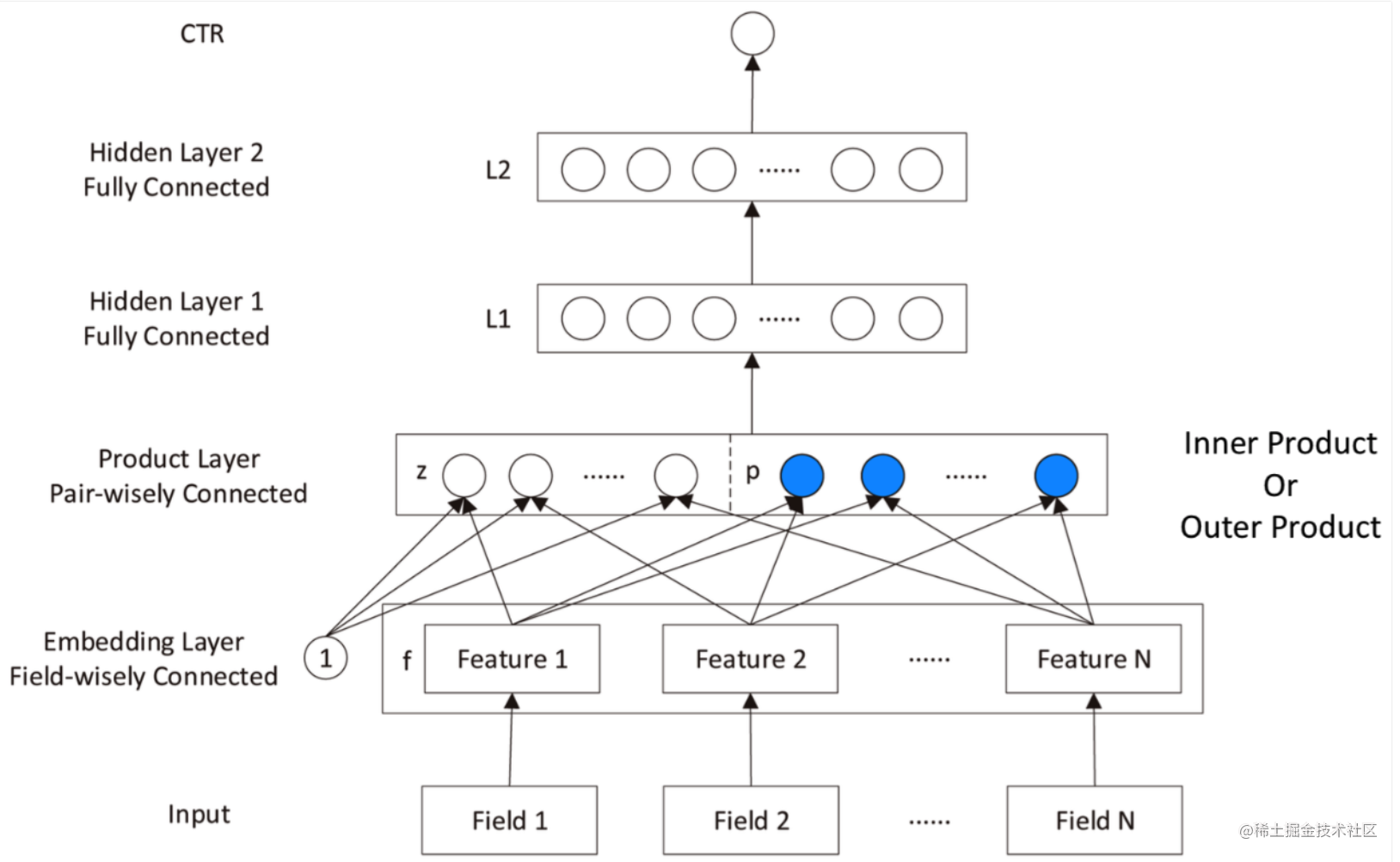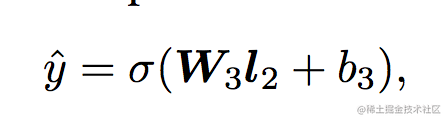l2层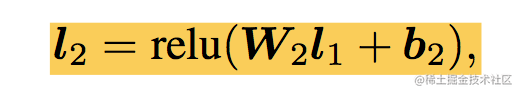l1层
l1层的输出由如下的公式计算：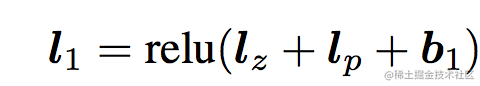Product Layer

product layer可以分成两个部分，一部分是线性部分lz，一部分是非线性部分lp。二者的形式如下：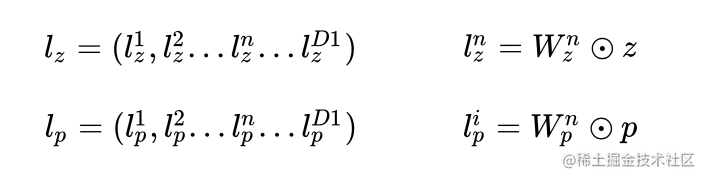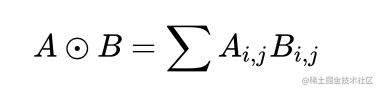Embedding Layer

Embedding Layer跟DeepFM中相同，将每一个field的特征转换成同样长度的向量，这里用f来表示。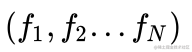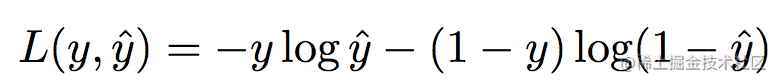# 2、Product Layer详细介绍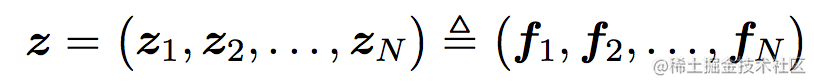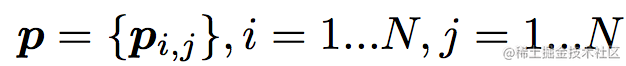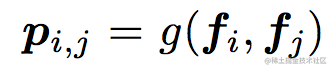## 2.1 IPNN

IPNN的示意图如下：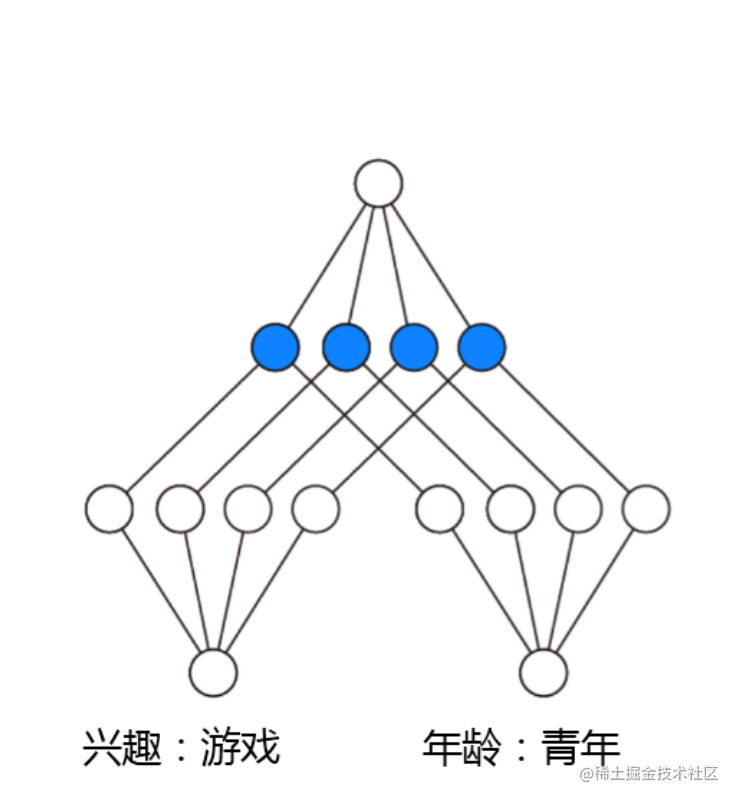IPNN中p的计算方式如下，即使用内积来代表pij：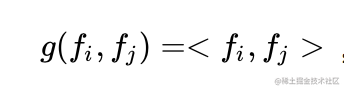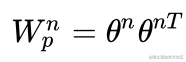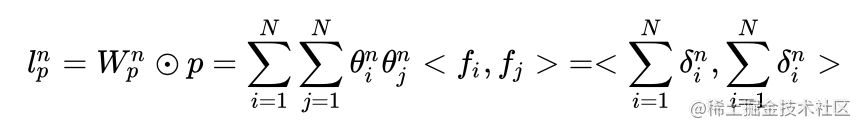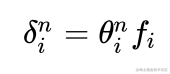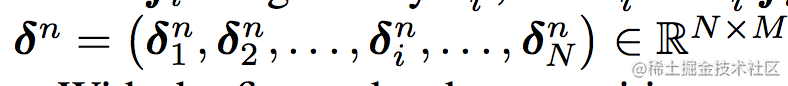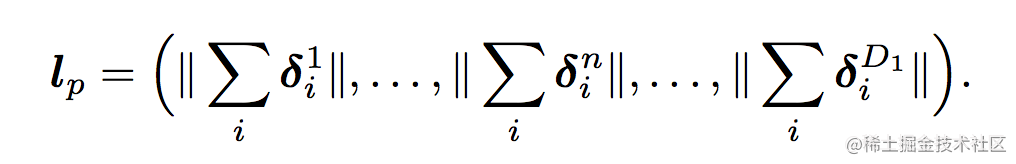## 2.2 OPNN

OPNN的示意图如下：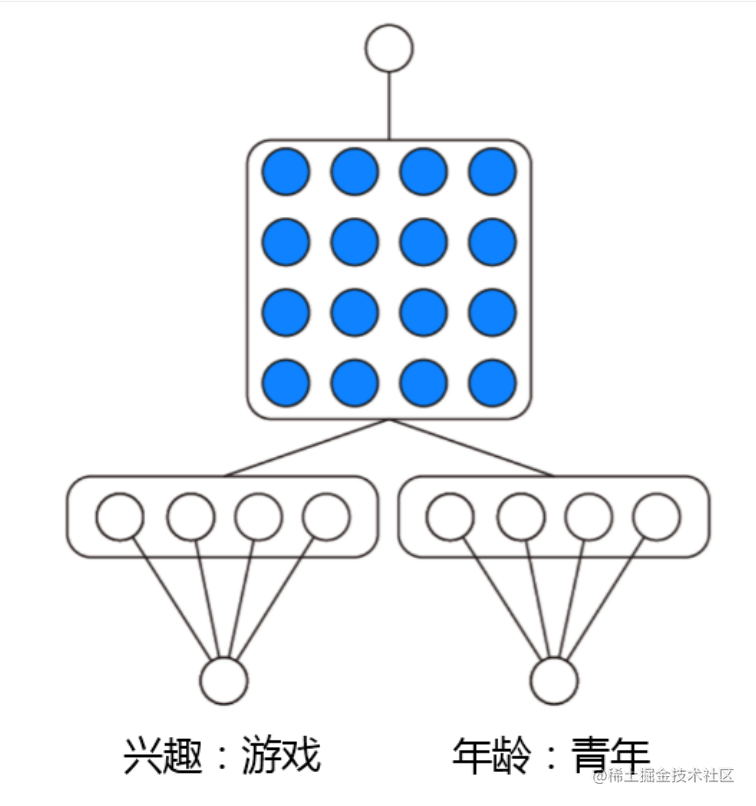OPNN中p的计算方式如下：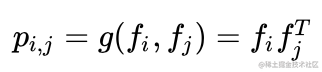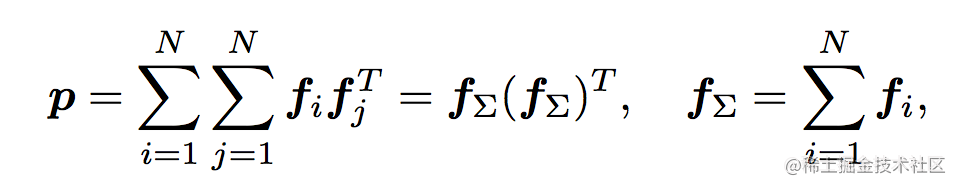# 3、代码实战

github.com/princewen/t….

``````self.feat_index = tf.placeholder(tf.int32,
shape=[None,None],
name='feat_index')
self.feat_value = tf.placeholder(tf.float32,
shape=[None,None],
name='feat_value')

self.label = tf.placeholder(tf.float32,shape=[None,1],name='label')
self.dropout_keep_deep = tf.placeholder(tf.float32,shape=[None],name='dropout_deep_deep')

feat_index是特征的一个序号，主要用于通过embedding_lookup选择我们的embedding。feat_value是对应的特征值，如果是离散特征的话，就是1，如果不是离散特征的话，就保留原来的特征值。label是实际值。还定义了dropout来防止过拟合。

``````def _initialize_weights(self):
weights = dict()

#embeddings
weights['feature_embeddings'] = tf.Variable(
tf.random_normal([self.feature_size,self.embedding_size],0.0,0.01),
name='feature_embeddings')
weights['feature_bias'] = tf.Variable(tf.random_normal([self.feature_size,1],0.0,1.0),name='feature_bias')

#Product Layers
if self.use_inner:
else:
tf.random_normal([self.deep_init_size, self.embedding_size,self.embedding_size], 0.0, 0.01))

weights['product-linear'] = tf.Variable(tf.random_normal([self.deep_init_size,self.field_size,self.embedding_size],0.0,0.01))
weights['product-bias'] = tf.Variable(tf.random_normal([self.deep_init_size,],0,0,1.0))
#deep layers
num_layer = len(self.deep_layers)
input_size = self.deep_init_size
glorot = np.sqrt(2.0/(input_size + self.deep_layers))

weights['layer_0'] = tf.Variable(
np.random.normal(loc=0,scale=glorot,size=(input_size,self.deep_layers)),dtype=np.float32
)
weights['bias_0'] = tf.Variable(
np.random.normal(loc=0,scale=glorot,size=(1,self.deep_layers)),dtype=np.float32
)

for i in range(1,num_layer):
glorot = np.sqrt(2.0 / (self.deep_layers[i - 1] + self.deep_layers[i]))
weights["layer_%d" % i] = tf.Variable(
np.random.normal(loc=0, scale=glorot, size=(self.deep_layers[i - 1], self.deep_layers[i])),
dtype=np.float32)  # layers[i-1] * layers[i]
weights["bias_%d" % i] = tf.Variable(
np.random.normal(loc=0, scale=glorot, size=(1, self.deep_layers[i])),
dtype=np.float32)  # 1 * layer[i]

glorot = np.sqrt(2.0/(input_size + 1))
weights['output'] = tf.Variable(np.random.normal(loc=0,scale=glorot,size=(self.deep_layers[-1],1)),dtype=np.float32)
weights['output_bias'] = tf.Variable(tf.constant(0.01),dtype=np.float32)

return weights

Embedding Layer

``````# Embeddings
self.embeddings = tf.nn.embedding_lookup(self.weights['feature_embeddings'],self.feat_index) # N * F * K
feat_value = tf.reshape(self.feat_value,shape=[-1,self.field_size,1])
self.embeddings = tf.multiply(self.embeddings,feat_value) # N * F * K

Product Layer

``````# Linear Singal
linear_output = []
for i in range(self.deep_init_size):
linear_output.append(tf.reshape(
tf.reduce_sum(tf.multiply(self.embeddings,self.weights['product-linear'][i]),axis=[1,2]),shape=(-1,1)))# N * 1

self.lz = tf.concat(linear_output,axis=1) # N * init_deep_size

# Quardatic Singal
if self.use_inner:
for i in range(self.deep_init_size):
theta = tf.multiply(self.embeddings,tf.reshape(self.weights['product-quadratic-inner'][i],(1,-1,1))) # N * F * K

else:
embedding_sum = tf.reduce_sum(self.embeddings,axis=1)
p = tf.matmul(tf.expand_dims(embedding_sum,2),tf.expand_dims(embedding_sum,1)) # N * K * K
for i in range(self.deep_init_size):
theta = tf.multiply(p,tf.expand_dims(self.weights['product-quadratic-outer'][i],0)) # N * K * K

self.lp = tf.concat(quadratic_output,axis=1) # N * init_deep_size

self.y_deep = tf.nn.dropout(self.y_deep, self.dropout_keep_deep)

Deep Part

``````# Deep component
for i in range(0,len(self.deep_layers)):